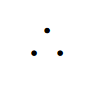How to write the symbol for therefore in LaTeX?

# How to write the symbol for therefore in LaTeX?

This post may contain affiliate links that allow us to earn a commission at no expense to you. Learn more

This article aims to show you the simplest and easiest way to write a symbol for therefore in LaTeX.

If you have worked with theorems in mathematics then you will know the therefore symbol and know how to use it. Today you will learn how to create it with LaTeX.

## Therefore Symbol

Use in logical arguments and mathematical proofs, this symbol or sign is usually used before a logical consequence, that means the conclusion. The symbols consist of 3 dots placed in a triangle shape.3 dots in an upright triangle represent the logical operator. Images created with LaTeX by the author.

## Therefore in LaTeX

LaTeX this time does not include by default the therefore symbol, so we will need to load the amssymb package which includes many symbols and one of them is this symbol in particular.

Once we have the package we will use the command \therefore inside math mode, to call the symbol. As you can see it does not require any argument or extra value of any kind.

For example

\documentclass{article}
\usepackage{amssymb} %we added the package to the document
\begin{document}
1) If words + words = sentences\\
$\therefore$ more words = more sentences
\\
\\
\vspace{5cm}
%another example
2) Let's say $x>$ 0, $\therefore\,$ $x \in \mathbb{N}$
\end{document}


The second example is one of the properties of the Natural numbers, the first example is a silly logical comment about words. Note: the “%” is used to comment inside the LaTeX code.

If you want to learn more about all the symbols the amssymb package can create, you can read the documentation as always. I hope this quick tutorial was helpful in guiding you using LaTeX, and as always keep writing in LaTeX.

All the images were created with LaTeX by the author.